# Maximum width of a binary tree

## Introduction

Before diving into the problem, let’s first understand the width or breadth of a binary tree. The width of a binary tree can be determined by the number of nodes present at the given level. To be more specific, the maximum number of nodes at any binary tree level is the maximum width of a binary tree.

Let’s understand it with some examples:

Example - 1: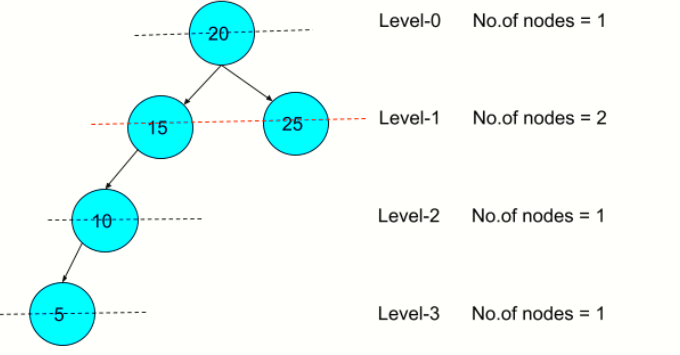Since the maximum number of nodes present at Level-1 is 2, the maximum width of the binary tree is 2

Example-2: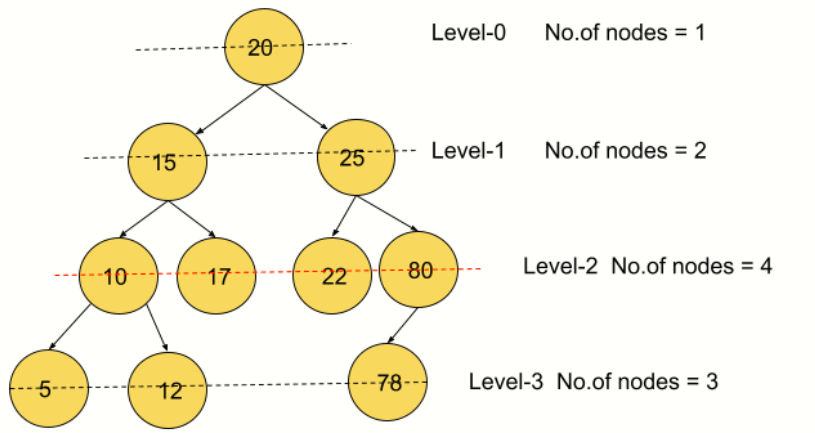As you can see, the maximum number of nodes present at level-2 is 4; hence the maximum width of the binary tree is 4.

Now that you know how to find the maximum width of a binary tree, we need to build an algorithm that will allow us to return the maximum width for any binary tree.

It is recommended to Implement the stated problem on your own before moving to the solution.

Let's discuss the approach to solve the stated problem:

## Method - Using Level-order Traversal

The naive yet effective approach can be using Level-wise traversal. As we aim to find the maximum number of nodes level-wise thus, employing Level order Traversal (or Breadth-first search) will suffice.

Level-order traversal or breadth-first search starts traversing at the tree from root and explores all nodes at the present level before moving on to the nodes at the next depth level.

Consider the below diagram:

Source: DEV Community

This is how the Level order traversal works in trees or any data structure.

Now let’s understand the algorithm for finding the maximum width of a binary tree using the Level-order traversal.

In the given binary tree:

Level 1 has 1 node, so maxWidth = 1

Level 2 has 2 node, so maxWidth = 2( 2 > 1)

Level 3 has 4 node, so maxWidth = 4( 4 > 2)

Hence, the maximum width will be 4.

### Algorithm:

1. Define the Node class, which contains three attributes: data, left, and right where, the left child of the node is represented by left, and the right child of the node is represented by right.
2. When a node is constructed, data is passed to the node's data attribute, and both left and right are set to null.
3. Create a new class with the root attribute.
Root represents the tree's root node and sets its value to null.
4. findMaxWidth() will find out the maximum width of a binary tree:

1. The variable maxWidth will keep track of the total number of nodes in each level.

2. The queue is used to traverse the binary tree on a level-by-level basis.

3. The function also determines if the root is null, indicating that the tree is empty.

4. If this is not the case, add the root node to the queue. Variable level_nodes keep track of the number of nodes in each level.

5. Remove the node from the front of the queue and add its left and right children to the queue if the number of level_nodes > 0.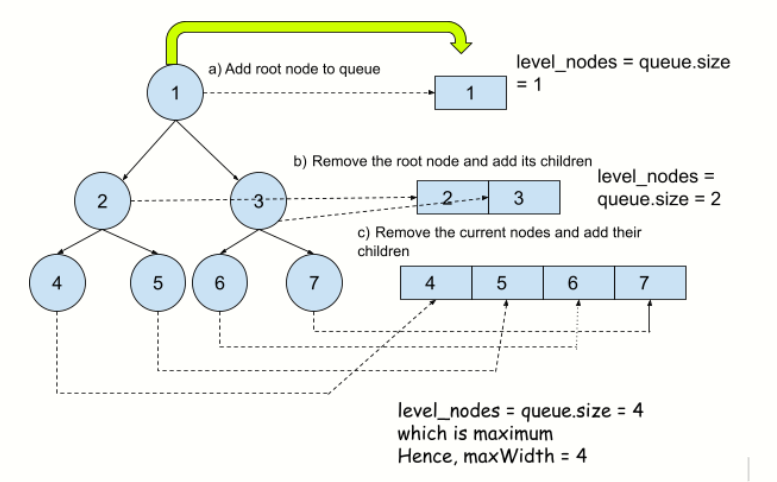In the above diagram, node 1 will be eliminated in the first iteration, and its children nodes 2 and 3 will be added to the queue. Node will be eliminated in the second iteration, and its offspring 4 and 5 will be added to the queue, and so on.

6. MaxWidth is a variable that stores the maximum width (maxWidth, level_nodes). As a result, it will always represent the maximum number of nodes at any given time.

5. Finally, Print the maxWidth.

Now, let’s look at the Implementation:

### Implementation in Java

Output

#### Complexity Analysis:

Time Complexity: O(N), where N is the number of nodes present in a binary tree. Since each node is processed once, therefore the time taken will be O(N).

Space Complexity: O(W), where W is the maximum width of a binary tree. Because, In level order traversal, a queue is kept whose maximum size at any given time can be as large as the binary tree's maximum width. In the worst case it can be O(N), where N is the number of nodes present in a binary tree.

### Implementation in python

Output

#### Complexity Analysis:

Time Complexity: O(N), where N is the number of nodes present in a binary tree. Since each node is processed once, therefore the time taken will be O(N).

Space Complexity: O(W), where W is the maximum width of a binary tree. Because, In level order traversal, a queue is kept whose maximum size at any given time can be as large as the binary tree's maximum width. In the worst case, it can be O(N), where N is the number of nodes present in a binary tree.

Pictorial Representation of the above example in implementation:-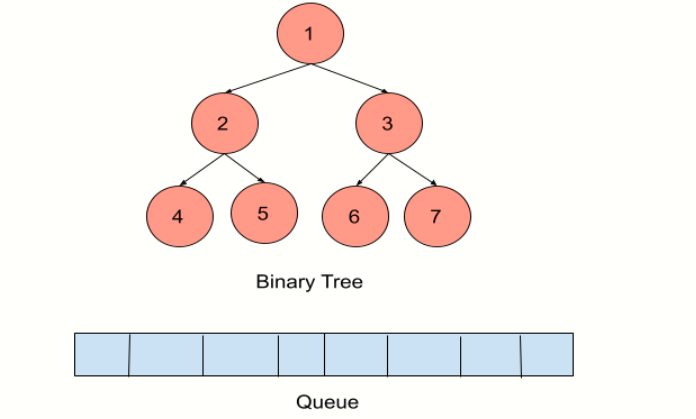Q1) What is the maximum width of a binary tree?

A1) The width of the binary tree is determined by the number of nodes in each level. As a result, the level with the most nodes will define the binary tree's maximum width.

Q2) What is the maximum number of nodes?

A2) If a binary tree has h levels, the maximum number of nodes is when all levels are filled.

The total number of nodes is 2^0 + 2^1 +.... 2^h = 2^(h+1)-1.

Q3) What is the height of a binary tree?

A3)The highest number of edges from the root to the farthest leaf node determines the height of a binary tree.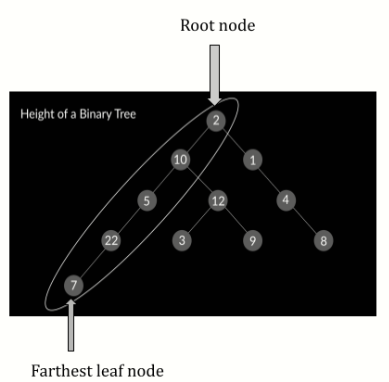Q4) What is the width of a binary tree?

A4) The number of nodes present at a given level determines the width of a binary tree.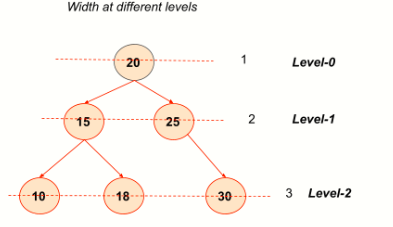Q5) What is the Level order traversal?

A5) As the name implies, level order traversal is a level by level traverse. We observed the width of a binary tree in the previous question, and in level order traversal, the search tree processes all the nodes in a tree by width, i.e., print all nodes of level 0 first, followed by nodes of level 1, and so on.

## Key takeaways

To summarise the session, we looked at finding the maximum width of a binary tree, which reflects the maximum number of nodes at any level. We've also looked at the intricacy of the proposed technique.

Don't sit idle; keep practising the most frequently asked questions to improve your learning and ensure a successful future.

Happy Learning Ninja!